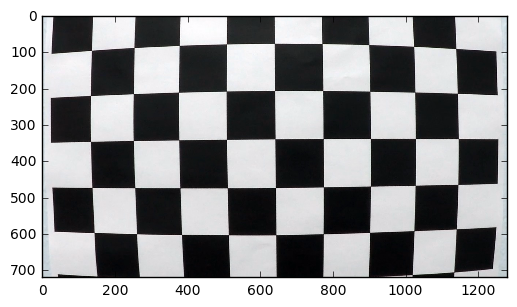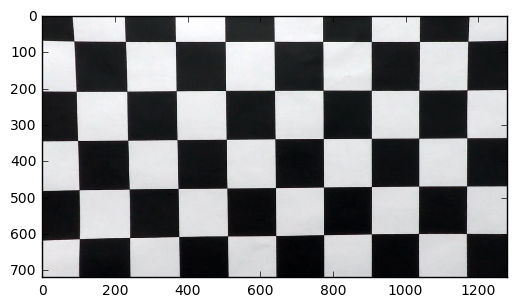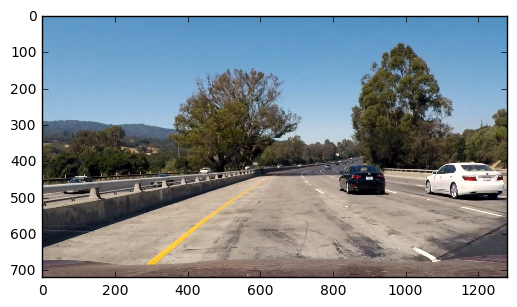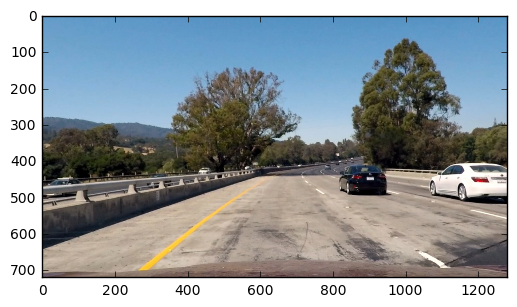In :
import numpy as np
import cv2
import glob
import matplotlib.pyplot as plt

# prepare object points, like (0,0,0), (1,0,0), (2,0,0) ....,(6,5,0)
objp = np.zeros((6*9,3), np.float32)
objp[:,:2] = np.mgrid[0:9,0:6].T.reshape(-1,2)

# Arrays to store object points and image points from all the images.
objpoints = [] # 3d points in real world space
imgpoints = [] # 2d points in image plane.

# Make a list of calibration images
images = glob.glob('./camera_cal/calibration*.jpg')

# Step through the list and search for chessboard corners
for fname in images:
gray = cv2.cvtColor(img,cv2.COLOR_BGR2GRAY)

# Find the chessboard corners
ret, corners = cv2.findChessboardCorners(gray, (9,6),None)

# If found, add object points, image points
if ret == True:
objpoints.append(objp)
imgpoints.append(corners)

In :
img = cv2.imread("./camera_cal/calibration1.jpg")
ret, mtx, dist, rvecs, tvecs = cv2.calibrateCamera(objpoints, imgpoints, (img.shape, img.shape),None,None)
undist = cv2.undistort(img, mtx, dist, None, mtx)

In :
%matplotlib inline
plt.imshow(img)

Out:
<matplotlib.image.AxesImage at 0x1169cbbe0>In :
plt.imshow(undist)

Out:
<matplotlib.image.AxesImage at 0x116df4f98>In :
img = plt.imread("./test_images/test1.jpg")
ret, mtx, dist, rvecs, tvecs = cv2.calibrateCamera(objpoints, imgpoints, (img.shape, img.shape),None,None)
undist = cv2.undistort(img, mtx, dist, None, mtx)

In :
plt.imshow(img)

Out:
<matplotlib.image.AxesImage at 0x11833d1d0>In :
plt.imshow(undist)

Out:
<matplotlib.image.AxesImage at 0x1108c6be0>In [ ]: